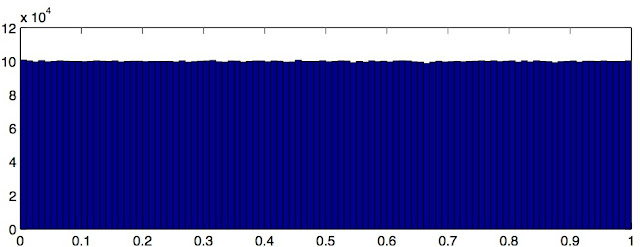## 2015/03/08

### Tinkering around logistic map

I was tinkering around logistic map $x_{n+1} = a x_n (1 - x_n)$ today and I wondered what happens if I plot the histogram of the generated sequence $(x_n)_{n\geq 0}$. Can it possess some statistical properties?

Logistic map is a well-known deterministic recurrence equation. Concisely, it is given as, \begin{align} x_{n+1} = a x_n (1 - x_n) \end{align} where $x_n \in (0,1)$. It is interesting because for $a = 4$, this system exhibits chaotic behaviour: Its response to a small uncertainty in the input is unpredictable. In other words, if you generate two sequences with very small difference in the initial condition, the sequences will differ vastly.

But as it turns out, it is not totally unpredictable. Let's see what we can always know for sure about this system. Take an initial condition $x_0 \in (0,1)$ (we assume we do not choose unstable points for now), and simulate $N$ steps. We have a sequence $(x_n)_{n=1}^N$. Treating these as samples, if you plot the histogram, you will get:This is true for any $x_0 \in (0,1)$ except for three unstable points $0.25, 0.5, 0.75$. Looks like a probability density. Indeed, Ulam and von Neumann found out that, if we see this sequence as a sequence of iid samples, the underlying probability density would be: \begin{align} p(x) = \frac{1}{\pi \sqrt{x(1-x)}} \end{align} More interestingly, it is possible to obtain uniform density by applying the following transformation: \begin{align} y_n = (2/\pi) \sin^{-1}(\sqrt{x_n}) \end{align}See the histogram where I used same samples with above after applying this transformation: